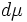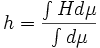# Average mean curvature

This article defines a notion of curvature for a differential manifold equipped with a Riemannian metric

This article defines a scalar value (viz, a real number) associated with a Riemannian manifold. This real number depends both on the underlying differential manifold and the Riemannian metric

## Definition

### Given data

A compact connected differential manifold$M$ with a Riemannian metric$g$.

### Definition part

The average mean curvature of$M$ is defined as the volume-averaged value of the mean curvature over the manifold. That is, if$H$ denotes the mean curvature and$d\mu$ the volume element, we have that:$h = \frac{\int H d\mu}{\int d\mu}$

Here, the volume element$d\mu$ is the natural choice of volume-element arising from the Riemannian metric.